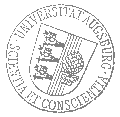# Universität AugsburgInstitut für MathematikOberseminar Differentialgeometrie

Dr. John Nicholson
Imperial College London

spricht am

Donnerstag, 20. Juli 2023

um

17:30 Uhr

im

Raum 2004 (L1)

über das Thema:

## »Simple homotopy types of 4-manifolds«

 Abstract: Two CW-complexes are simple homotopy equivalent if they are related by a sequence of collapses and expansions of cells. This notion interpolates between homeomorphism and homotopy in the sense that simple homotopy equivalent implies homotopy equivalent, and homeomorphic implies simple homotopy equivalent. It consequently proved extremely useful in manifold topology and is behind the s-cobordism theorem which is the basis for the vast majority of manifold classification results in dimension at least 4. The aim of this talk will be to present the first examples of two 4-manifolds which are homotopy equivalent but not simple homotopy equivalent, as well as in all higher even dimensions. The examples are constructed using surgery theory and the s-cobordism theorem, and are distinguished using methods from algebraic number theory and algebraic K-theory. This is joint work with Csaba Nagy and Mark Powell.

 Hierzu ergeht herzliche Einladung. Prof. Dr. Bernhard Hanke

Kaffee, Tee und Gebäck eine halbe Stunde vor Vortragsbeginn im Raum 2006 (L1).

[Impressum]      [Datenschutz]      wwwadm@math.uni-augsburg.de,     Di 11-Jul-2023 13:44:40 MESZ